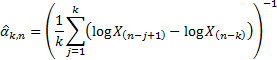/

### Enterprise Risk Management Formula Book 11. Extreme value theory

11.1        Maximum domain of attraction (MDA)

Suppose that i.i.d. random variables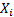have cdf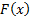. Suppose also that there exist sequences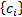and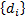and a cdf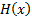such that: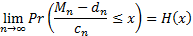where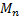is the random variable corresponding to the block maximum for blocks of such variables of length, i.e. each (independent) realisation of the series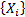is used to create a realisation ofgiven by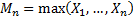.

Then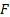is said to be in the maximum domain of attraction (MDA) of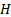, written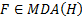11.2        Fisher-Tippett theorem

Ifwhereis a non-degenerate cdf thenmust be a Generalised Extreme Value (GEV) distribution.

If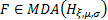where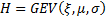then by replacing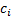by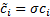and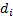by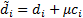we see that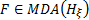where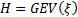.

11.3        The Pickands-Balkema-de Haan (PBH) theorem

Let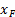be the maximum limiting value of the random variable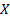. Then the PBH theorem states that we can find a function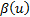such that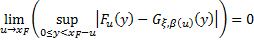if and only if that11.4        Estimating tail distributions

Suppose that the underlying loss distribution is in the maximum domain of attraction of the Frechét distribution and it has a tail of the form: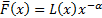for some slowly varying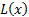, where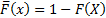. Then the Hill estimator for the (upper) tail index, givenordered observations,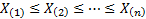, assuming that the (upper) tail contains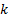entries is: